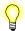# clock()

Return the number of clock ticks used by the program

## Synopsis:

```#include <time.h>

clock_t clock( void );```

## Library:

libc

Use the -l c option to qcc to link against this library. This library is usually included automatically.

## Description:

The clock() function returns the number of clock ticks of processor time used by the program since it started executing. You can convert the number of ticks into seconds by dividing by the value CLOCKS_PER_SEC.In a multithreaded program, clock() returns the time used by all threads in the application; clock() returns the time since the program started, not the time since a specific thread started.

## Returns:

The number of clock ticks, or (clock_t) -1 if the number of ticks couldn't be determined or exceeds the maximum value that the clock_t type can represent.

## Examples:

```#include <stdio.h>
#include <math.h>
#include <time.h>
#include <stdlib.h>

void compute( void )
{
int i, j;
double x;

x = 0.0;
for( i = 1; i <= 100; i++ ) {
for( j = 1; j <= 100; j++ ) {
x += sqrt( (double) i * j );
}
}

printf( "%16.7f\n", x );
}

int main( void )
{
clock_t start_time, end_time;

start_time = clock();
compute();
end_time = clock();
printf( "Execution time was %lu seconds\n",
(long) ((end_time - start_time) / CLOCKS_PER_SEC) );

return EXIT_SUCCESS;
}```

## Classification:

Safety:
Cancellation point No
Interrupt handler No
Signal handler Yes